购物车 (0)EA分类
• 黑盒B-Box
统计套利策略
统计套利策略
• 极影机器人
网格策略，盈利稳定
网格策略，盈利稳定
• 原油碰撞机
原油策略，经典对冲
原油策略，经典对冲
• 头皮风暴
头皮策略，闪电交易
头皮策略，闪电交易
• 百元EA
西点延迟EA（带Lmax账号）海龟趋势加仓EA评测
西点延迟EA（带Lmax账号）
海龟趋势加仓
EA评测
• 免费工具
跟单EA28货币EA切换MT4背景
跟单EA
28货币EA
切换MT4背景
• EA定制和VPS租用
EA定制，修改，VPS租用VPS租用服务
EA定制，修改，VPS租用
VPS租用服务

# 海龟汤和海龟汤升级版的改进

### 简介

《华尔街智慧：高胜算短线交易策略》一书的作者，劳伦斯.康纳斯和琳达.瑞斯克是有着34年交易经验的成功交易者，他们的经验包括股票交易，以及在银行、对冲基金、经济公司和咨询公司的职位。他们相信，您只需要一个交易策略就能够做到稳定获利地交易。但是，书中还是包含了20种左右不同的交易策略，分成四组，每组针对不同的市场周期，并且运用于一种稳定的价格行为模式。

### ‘海龟汤’交易系统与‘海龟汤升级版’的改进

1. 确认距离前一个20天低点至少过去了3天

2. 等待资产价格跌破20天低点

3. 在向下突破的价格低点上方5到10个点设置买入挂单

4. 当挂单触发时，把止损设于当日最低价下方一个点的位置

5. 当仓位有利润后使用跟踪止损

6. 如果仓位在第一天或者第二天由止损关闭，您可以在初始水平重复进场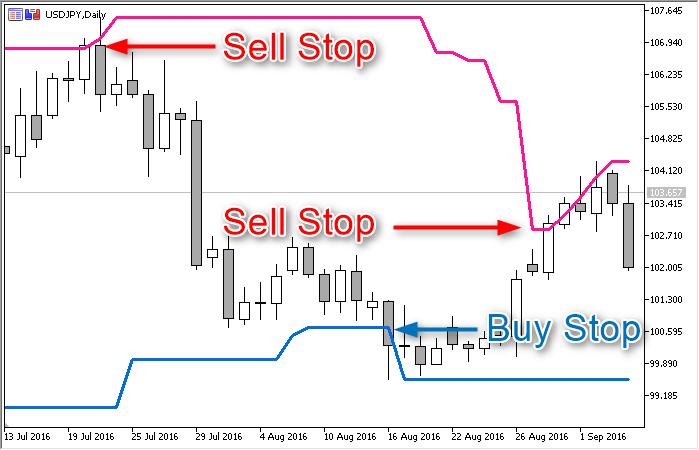1. 不是在突破20天范围后立即设置挂单，而是等待一个确认信号 - 当天的柱收盘于范围之外，当日收盘在分析所得的水平通道边界之外也是可以的。

2. 为了确定初始止损水平，我们使用两天的极值（最高或者最低价）水平。

### 定义通道参数

```struct CHANNEL {
double    d_High;           // 范围上方边界的价格
double    d_Low;            // 范围下方边界的价格
datetime  t_From;           // 通道中第一个（最早的）柱的日期/时间
datetime  t_To;             // 通道中最后一个柱的日期/时间
int       i_Highest_Offset; // 最高价右边的柱数
int       i_Lowest_Offset;  // 最低价右边的柱数
};

```

```void f_Set(int i_Bars_Limit, int i_Newest_Bar_Shift = 1) {
double da_Price_Array[]; // 用于保存通道中所有柱的最高/最低价格的辅助数组

// 确定通道的上方边界:

int i_Price_Bars = CopyHigh(_Symbol, PERIOD_CURRENT, i_Newest_Bar_Shift, i_Bars_Limit, da_Price_Array);
int i_Bar = ArrayMaximum(da_Price_Array);
d_High = da_Price_Array[i_Bar]; // 确定通道的上方边界
i_Highest_Offset = i_Price_Bars - i_Bar; // 距离最高价的时间(柱数)

// 确定范围的最低边界:

i_Price_Bars = CopyLow(_Symbol, PERIOD_CURRENT, i_Newest_Bar_Shift, i_Bars_Limit, da_Price_Array);
i_Bar = ArrayMinimum(da_Price_Array);
d_Low = da_Price_Array[i_Bar]; // 确定通道的最低边界
i_Lowest_Offset = i_Price_Bars - i_Bar; // 距离最低点的时间(柱数)

datetime ta_Time_Array[];
i_Price_Bars = CopyTime(_Symbol, PERIOD_CURRENT, i_Newest_Bar_Shift, i_Bars_Limit, ta_Time_Array);
t_From = ta_Time_Array;
t_To = ta_Time_Array[i_Price_Bars - 1];
}

```

```enum ENUM_LOG_LEVEL { // 记录级别
LOG_LEVEL_NONE,     // 禁用记录
LOG_LEVEL_ERR,      // 只含有错误信息
LOG_LEVEL_INFO,     // 错误 + EA的注释
LOG_LEVEL_DEBUG     // 所有内容
};

```

```void f_Set(int i_Bars_Limit, int i_Newest_Bar_Shift = 1) {
double da_Price_Array[]; // 用于保存通道中所有柱的最高/最低价格的辅助数组

// 确定通道的上方边界:

int i_Price_Bars = CopyHigh(_Symbol, PERIOD_CURRENT, i_Newest_Bar_Shift, i_Bars_Limit, da_Price_Array);

if(i_Price_Bars == WRONG_VALUE) {    // 处理 CopyHigh 函数的错误    if(Log_Level > LOG_LEVEL_NONE) PrintFormat("%s: CopyHigh: 错误 #%u", __FUNCSIG__, _LastError);    return;   }    if(i_Price_Bars < i_Bars_Limit) {    // CopyHigh 函数未能接收到请求数量的数据    if(Log_Level > LOG_LEVEL_NONE) PrintFormat("%s: CopyHigh: 已经复制了 %u 个柱，共需 %u", __FUNCSIG__, i_Price_Bars, i_Bars_Limit);    return;   }
int i_Bar = ArrayMaximum(da_Price_Array);
if(i_Bar == WRONG_VALUE) {    // 处理 ArrayMaximum 函数的错误    if(Log_Level > LOG_LEVEL_NONE) PrintFormat("%s: ArrayMaximum: 错误 #%u", __FUNCSIG__, _LastError);    return;   }
d_High = da_Price_Array[i_Bar]; // 确定通道的上方边界
i_Highest_Offset = i_Price_Bars - i_Bar; // 距离最高价的时间(柱数)

// 确定范围的最低边界:

i_Price_Bars = CopyLow(_Symbol, PERIOD_CURRENT, i_Newest_Bar_Shift, i_Bars_Limit, da_Price_Array);

if(i_Price_Bars == WRONG_VALUE) {    // 处理 CopyLow 函数的错误    if(Log_Level > LOG_LEVEL_NONE) PrintFormat("%s: CopyLow: 错误 #%u", __FUNCSIG__, _LastError);    return;   }    if(i_Price_Bars < i_Bars_Limit) {    // CopyLow 函数未能收到所请求数量的数据    if(Log_Level > LOG_LEVEL_NONE) PrintFormat("%s: CopyLow: 已复制 %u 个柱，共需 %u", __FUNCSIG__, i_Price_Bars, i_Bars_Limit);    return;   }
i_Bar = ArrayMinimum(da_Price_Array);
if(i_Bar == WRONG_VALUE) {    // 处理 ArrayMinimum 函数的错误    if(Log_Level > LOG_LEVEL_NONE) PrintFormat("%s: ArrayMinimum: 错误 #%u", __FUNCSIG__, _LastError);    return;   }  d_Low = da_Price_Array[i_Bar]; // 确定通道的最低边界
i_Lowest_Offset = i_Price_Bars - i_Bar; // 距离最低点的时间(柱数)

datetime ta_Time_Array[];
i_Price_Bars = CopyTime(_Symbol, PERIOD_CURRENT, i_Newest_Bar_Shift, i_Bars_Limit, ta_Time_Array);
if(i_Price_Bars < 1) t_From = t_To = 0;
else {
t_From = ta_Time_Array;
t_To = ta_Time_Array[i_Price_Bars - 1];
}
}

```

```// 用于在一个结构中收集和更新通道的信息和函数struct CHANNEL {
// 变量
double    d_High;           // 范围上方边界的价格
double    d_Low;            // 范围下方边界的价格
datetime  t_From;           // 通道的第一个（最前面的）柱的日期/时间
datetime  t_To;             // 通道中最后一个柱的日期/时间
int       i_Highest_Offset; // 最高价右边的柱数
int       i_Lowest_Offset;  // 最低价右边的柱数
bool      b_Updated;        // 通道参数改变了吗?
string    s_Signature;      // 最后数据的签名

// 函数:

CHANNEL() {    d_High = d_Low = 0;    t_From = t_To = 0;    b_Ready = b_Updated = false;    s_Signature = "-";    i_Highest_Offset = i_Lowest_Offset = WRONG_VALUE;   }
void f_Set(int i_Bars_Limit, int i_Newest_Bar_Shift = 1) {
b_Ready = false; // Pitstop: 设置一个服务标志
double da_Price_Array[]; // 用于保存通道中所有柱的最高/最低价格的辅助数组

// 确定通道的上方边界:

int i_Price_Bars = CopyHigh(_Symbol, PERIOD_CURRENT, i_Newest_Bar_Shift, i_Bars_Limit, da_Price_Array);
if(i_Price_Bars == WRONG_VALUE) {
// 处理 CopyHigh 函数的错误
if(Log_Level > LOG_LEVEL_NONE) PrintFormat("%s: CopyHigh: 错误 #%u", __FUNCSIG__, _LastError);
return;
}

if(i_Price_Bars < i_Bars_Limit) {
// CopyHigh 函数未能接收到请求数量的数据
if(Log_Level > LOG_LEVEL_NONE) PrintFormat("%s: CopyHigh: 已经复制了 %u 个柱，共需 %u", __FUNCSIG__, i_Price_Bars, i_Bars_Limit);
return;
}

int i_Bar = ArrayMaximum(da_Price_Array);
if(i_Bar == WRONG_VALUE) {
// 处理 ArrayMaximum 函数的错误
if(Log_Level > LOG_LEVEL_NONE) PrintFormat("%s: ArrayMaximum: 错误 #%u", __FUNCSIG__, _LastError);
return;
}

d_High = da_Price_Array[i_Bar]; // 确定通道的上方边界
i_Highest_Offset = i_Price_Bars - i_Bar; // 距离最高价的时间(柱数)

// 确定范围的最低边界:

i_Price_Bars = CopyLow(_Symbol, PERIOD_CURRENT, i_Newest_Bar_Shift, i_Bars_Limit, da_Price_Array);

if(i_Price_Bars == WRONG_VALUE) {
// 处理 CopyLow 函数的错误
if(Log_Level > LOG_LEVEL_NONE) PrintFormat("%s: CopyLow: 错误 #%u", __FUNCSIG__, _LastError);
return;
}

if(i_Price_Bars < i_Bars_Limit) {
// CopyLow 函数未能收到所请求数量的数据
if(Log_Level > LOG_LEVEL_NONE) PrintFormat("%s: CopyLow: 已复制 %u 个柱，共需 %u", __FUNCSIG__, i_Price_Bars, i_Bars_Limit);
return;
}

i_Bar = ArrayMinimum(da_Price_Array);
if(i_Bar == WRONG_VALUE) {
// 处理 ArrayMinimum 函数的错误
if(Log_Level > LOG_LEVEL_NONE) PrintFormat("%s: ArrayMinimum: 错误 #%u", __FUNCSIG__, _LastError);
return;
}
d_Low = da_Price_Array[i_Bar]; // 确定通道的最低边界
i_Lowest_Offset = i_Price_Bars - i_Bar; // 距离最低点的时间(柱数)

datetime ta_Time_Array[];
i_Price_Bars = CopyTime(_Symbol, PERIOD_CURRENT, i_Newest_Bar_Shift, i_Bars_Limit, ta_Time_Array);
if(i_Price_Bars < 1) t_From = t_To = 0;
else {
t_From = ta_Time_Array;
t_To = ta_Time_Array[i_Price_Bars - 1];
}

string s_New_Signature = StringFormat("%.5f%.5f%u%u", d_Low, d_High, t_From, t_To);    if(s_Signature != s_New_Signature) {      // 通道数据已经改变      b_Updated = true;      if(Log_Level > LOG_LEVEL_ERR) PrintFormat("%s: 通道已更新: %s .. %s / %s .. %s, min: %u max: %u ", __FUNCTION__, DoubleToString(d_Low, _Digits), DoubleToString(d_High, _Digits), TimeToString(t_From, TIME_DATE|TIME_MINUTES), TimeToString(t_To, TIME_DATE|TIME_MINUTES), i_Lowest_Offset, i_Highest_Offset);      s_Signature = s_New_Signature;    }
b_Ready = true; // 数据更新成功完成  }
};

```

`CHANNEL go_Channel;`

### 信号生成函数

1. 距离前一个20天低点至少已经过了3个交易日

2a. 交易品种的价格低于20天低点 (海龟汤)

2b. 每日柱的收盘价不高于20天的低点 (海龟汤升级版)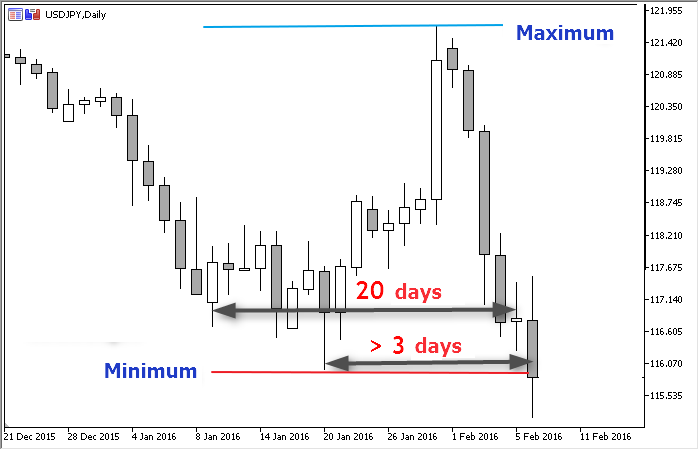```enum ENUM_STRATEGY {     // 策略列表
TS_TURTLE_SOUP,        // 海龟汤
TS_TURTLE_SOUP_PLUS_1  // 海龟汤升级版
};
input ENUM_STRATEGY  Strategy_Type = TS_TURTLE_SOUP;  // 交易策略:```

```enum ENUM_ENTRY_SIGNAL {  // 进场信号的列表
ENTRY_SELL,             // 卖出信号
ENTRY_NONE,             // 没有信号
ENTRY_UNKNOWN           // 未定义状态
};

```

`MqlTick go_Tick; // 最后所知订单时刻的信息`

```ENUM_ENTRY_SIGNAL fe_Get_Entry_Signal(
bool b_Wait_For_Bar_Close = false,
int i_Extremum_Bars = 3
) {}

```

```if(go_Channel.i_Highest_Offset > i_Extremum_Bars) // 第一个条件
if(go_Channel.d_High < d_Actual_Price) // 第二个条件
return(ENTRY_SELL); // 两个卖出条件都已达到```

```if(go_Channel.i_Lowest_Offset > i_Extremum_Bars) // 第一个条件
if(go_Channel.d_Low > d_Actual_Price) { // 第二个条件

```double d_Actual_Price = go_Tick.bid; // 默认价格 - 用于海龟汤版本if(b_Wait_For_Bar_Close) { // 用于海龟汤升级版版本
double da_Price_Array; // 辅助数组
CopyClose(_Symbol, PERIOD_CURRENT, 1, 1, da_Price_Array));
d_Actual_Price = da_Price_Array;
}

```

```ENUM_ENTRY_SIGNAL fe_Get_Entry_Signal(bool b_Wait_For_Bar_Close = false, int i_Extremum_Bars = 3) {
double d_Actual_Price = go_Tick.bid; // 默认价格 - 用于海龟汤版本
if(b_Wait_For_Bar_Close) { // 用于海龟汤升级版版本
double da_Price_Array;
CopyClose(_Symbol, PERIOD_CURRENT, 1, 1, da_Price_Array));
d_Actual_Price = da_Price_Array;
}

// 上方边界:
if(go_Channel.i_Highest_Offset > i_Extremum_Bars) // 第一个条件
if(go_Channel.d_High < d_Actual_Price) { // 2nd condition
// 价格突破了上方边界
return(ENTRY_SELL);
}

// 下方边界:
if(go_Channel.i_Lowest_Offset > i_Extremum_Bars) // 第一个条件
if(go_Channel.d_Low > d_Actual_Price) { // 第二个条件
// 价格突破了下方边界
}

return(ENTRY_NONE);
}

```

```ENUM_ENTRY_SIGNAL fe_Get_Entry_Signal(bool b_Wait_For_Bar_Close = false, int i_Extremum_Bars = 3) {
if(!go_Channel.b_Ready) {    // 通道数据还没有准备好    if(Log_Level == LOG_LEVEL_DEBUG) PrintFormat("%s: 通道参数没有准备好", __FUNCTION__);    return(ENTRY_UNKNOWN);   }
double d_Actual_Price = go_Tick.bid; // 默认价格 - 用于海龟汤版本
if(b_Wait_For_Bar_Close) { // 用于海龟汤升级版版本
double da_Price_Array;
if(WRONG_VALUE == CopyClose(_Symbol, PERIOD_CURRENT, 1, 1, da_Price_Array)) {      // 处理 CopyClose 函数的错误      if(Log_Level > LOG_LEVEL_NONE) PrintFormat("%s: CopyClose: 错误 #%u", __FUNCSIG__, _LastError);      return(ENTRY_NONE);    }    d_Actual_Price = da_Price_Array;
}

// 上方边界:
if(go_Channel.i_Highest_Offset > i_Extremum_Bars) // 第一个条件
if(go_Channel.d_High < d_Actual_Price) { // 2nd condition
// 价格突破了上方边界      if(Log_Level == LOG_LEVEL_DEBUG) PrintFormat("%s: 价格 (%s) 已经突破了上方边界 (%s)", __FUNCTION__, DoubleToString(d_Actual_Price, _Digits), DoubleToString(go_Channel.d_High, _Digits));      return(ENTRY_SELL);
}

// 下方边界:
if(go_Channel.i_Lowest_Offset > i_Extremum_Bars) // 第一个条件
if(go_Channel.d_Low > d_Actual_Price) { // 第二个条件
// 价格突破了下方边界      if(Log_Level == LOG_LEVEL_DEBUG) PrintFormat("%s: 价格 (%s) 已经突破了下方边界 (%s)", __FUNCTION__, DoubleToString(d_Actual_Price, _Digits), DoubleToString(go_Channel.d_Low, _Digits));      return(ENTRY_BUY);
}

// 如果程序达到了这一行，价格就在范围之内，也就是第二个条件不满足

return(ENTRY_NONE);
}

```

```ENUM_ENTRY_SIGNAL fe_Get_Entry_Signal(bool b_Wait_For_Bar_Close = false, int i_Extremum_Bars = 3) {
staticdatetime st_Pause_End = 0; // 下一次检查的时间   if(st_Pause_End > go_Tick.time) return(ENTRY_NONE);   st_Pause_End = 0;
if(go_Channel.b_In_Process) {
// 通道数据还没有准备好
if(Log_Level == LOG_LEVEL_DEBUG) PrintFormat("%s: 通道参数没有准备好", __FUNCTION__);
return(ENTRY_UNKNOWN);
}
if(go_Channel.i_Lowest_Offset < i_Extremum_Bars && go_Channel.i_Highest_Offset < i_Extremum_Bars) {    // 第一个条件不满足    if(Log_Level == LOG_LEVEL_DEBUG) PrintFormat("%s: 第一个条件不满足", __FUNCTION__);        // 暂停，直到通道更新    st_Pause_End = go_Tick.time + PeriodSeconds() - go_Tick.time % PeriodSeconds();        return(ENTRY_NONE);   }
double d_Actual_Price = go_Tick.bid; // 默认价格 - 用于海龟汤版本
if(b_Wait_For_Bar_Close) { // 用于海龟汤升级版版本
double da_Price_Array;
if(WRONG_VALUE == CopyClose(_Symbol, PERIOD_CURRENT, 1, 1, da_Price_Array)) {
// 处理 CopyClose 函数的错误
if(Log_Level > LOG_LEVEL_NONE) PrintFormat("%s: CopyClose: 错误 #%u", __FUNCSIG__, _LastError);
return(ENTRY_NONE);
}
d_Actual_Price = da_Price_Array;
}

// 上方边界:
if(go_Channel.i_Highest_Offset > i_Extremum_Bars) // 第一个条件
if(go_Channel.d_High < d_Actual_Price) { // 2nd condition
// 价格突破了上方边界
if(Log_Level == LOG_LEVEL_DEBUG) PrintFormat("%s: 价格 (%s) 已经突破了上方边界 (%s)", __FUNCTION__, DoubleToString(d_Actual_Price, _Digits), DoubleToString(go_Channel.d_High, _Digits));
return(ENTRY_SELL);
}

// 下方边界:
if(go_Channel.i_Lowest_Offset > i_Extremum_Bars) // 第一个条件
if(go_Channel.d_Low > d_Actual_Price) { // 第二个条件
// 价格突破了下方边界
if(Log_Level == LOG_LEVEL_DEBUG) PrintFormat("%s: 价格 (%s) 已经突破了下方边界 (%s)", __FUNCTION__, DoubleToString(d_Actual_Price, _Digits), DoubleToString(go_Channel.d_Low, _Digits));
}

// 如果程序达到了这一行，价格就在范围之内，也就是第二个条件不满足

if(b_Wait_For_Bar_Close) // 对于海龟汤升级版    // 暂停，直到当前柱关闭    st_Pause_End = go_Tick.time + PeriodSeconds() - go_Tick.time % PeriodSeconds();
return(ENTRY_NONE);
}

```

```enum ENUM_STRATEGY {     // 策略版本
TS_TURTLE_SOUP,        // 海龟汤
TS_TURTLE_SOUP_PLIS_1  // 海龟汤升级版
};

// 自定义设置input ENUM_STRATEGY  Turtle_Soup_Type = TS_TURTLE_SOUP;  // 海龟汤: 策略版本inputuint           Turtle_Soup_Period_Length = 20;     // 海龟汤: 极值点搜索深度 (柱数)inputuint           Turtle_Soup_Extremum_Offset = 3;    // 海龟汤: 距离上一个极值点的暂停(柱数)inputdouble         Turtle_Soup_Entry_Offset = 10;      // 海龟汤: 进场: 与极值水平的偏移 (点数)inputdouble         Turtle_Soup_Exit_Offset = 1;        // 海龟汤: 推出: 与相反极值点的偏移 (点数)```

### 用于测试交易策略的基础EA交易

```enum ENUM_LOG_LEVEL {  // 记录级别列表
LOG_LEVEL_NONE,      // 记录被禁用
LOG_LEVEL_ERR,       // 只记录错误信息
LOG_LEVEL_INFO,      // 错误 + EA的注释
LOG_LEVEL_DEBUG      // 所有内容
};
enum ENUM_ENTRY_SIGNAL {  // 进场信号的列表
ENTRY_SELL,             // 卖出信号
ENTRY_NONE,             // 没有信号
ENTRY_UNKNOWN           // 未定义状态
};

#include <Trade\Trade.mqh> // 用于进行交易操作的类inputstring  _ = "** 策略设置:";  // .#include <Expert\Signal\Signal_Turtle_Soup.mqh> //信号模块

inputstring  __ = "** 仓位建立和管理:"; // .inputdouble  Trade_Volume = 0.1;                  // 交易量inputuint    Trail_Trigger = 100;                 // 移动止损: 距离跟踪的点数

```int
gi_Connect_Wait = 2000// 在尝试前的暂停时间(毫秒数)
;
double
gd_Stop_Level, // 把止损水平根据设置转换为交易品种价格
gd_Lot_Step, gd_Lot_Min, gd_Lot_Max, // 手数限制的设置   gd_Entry_Offset, // 进场: 距离极值点的交易品种价格距离   gd_Exit_Offset, // 退出: 交易品种价格中与极值点的偏移   gd_Trail_Trigger, gd_Trail_Step, gd_Trail_Distance // 转换为交易品种价格的跟踪止损参数;MqlTick go_Tick; // 最后所知订单时刻的信息intOnInit() {   // 把设置从点数转换为交易品种价格:   double d_One_Point_Rate = pow(10, _Digits);   gd_Entry_Offset = Turtle_Soup_Entry_Offset / d_One_Point_Rate;   gd_Exit_Offset = Turtle_Soup_Exit_Offset / d_One_Point_Rate;   gd_Trail_Trigger = Trail_Trigger / d_One_Point_Rate;   gd_Trail_Step = Trail_Step / d_One_Point_Rate;   gd_Trail_Distance = Trail_Distance / d_One_Point_Rate;   gd_Stop_Level = SymbolInfoInteger(_Symbol, SYMBOL_TRADE_STOPS_LEVEL) / d_One_Point_Rate;   // 手数限制的初始化:   gd_Lot_Min = SymbolInfoDouble(_Symbol, SYMBOL_VOLUME_MIN);   gd_Lot_Max = SymbolInfoDouble(_Symbol, SYMBOL_VOLUME_MAX);   gd_Lot_Step = SymbolInfoDouble(_Symbol, SYMBOL_VOLUME_STEP);    return(INIT_SUCCEEDED);}```

```bool fb_Trailing_Stop(    // 当前交易品种仓位的跟踪止损
double d_Trail_Trigger,  // 启用跟踪止损的距离
double d_Trail_Step,    // SL 跟踪止损步长 (以交易品种价格计算)
double d_Trail_Distance  // 价格距离止损的最小距离 (在交易品种价格中)</s3>) {   if(!PositionSelect(_Symbol)) return(false); // 没有仓位，不需要跟踪止损    // 计算新的止损水平的基础数值 - 当前价格数值:   double d_New_SL = PositionGetDouble(POSITION_PRICE_CURRENT);    if(PositionGetInteger(POSITION_TYPE) == POSITION_TYPE_BUY) { // 对于买入仓位    if(d_New_SL - PositionGetDouble(POSITION_PRICE_OPEN) < d_Trail_Trigger)      return(false); // 价格没有移动到足够的位置来启用跟踪止损          if(d_New_SL - PositionGetDouble(POSITION_SL) < d_Trail_Distance + d_Trail_Step)      return(false); // 价格改动少于设置的跟踪止损步长        d_New_SL -= d_Trail_Distance; // 新的止损水平   } elseif(PositionGetInteger(POSITION_TYPE) == POSITION_TYPE_SELL) { // 对于卖出仓位    if(PositionGetDouble(POSITION_PRICE_OPEN) - d_New_SL < d_Trail_Trigger)      return(false); // 价格没有移动到足够的位置来启用跟踪止损        if(PositionGetDouble(POSITION_SL) > 0.0) if(PositionGetDouble(POSITION_SL) - d_New_SL < d_Trail_Distance + d_Trail_Step)      return(false); // 价格没有移动到足够的位置来启用跟踪止损        d_New_SL += d_Trail_Distance; // New SL level   } elsereturn(false);    // 服务器设置是否允许在与当前价格这样的距离中设置止损?   if(!fb_Is_Acceptable_Distance(d_New_SL, PositionGetDouble(POSITION_PRICE_CURRENT))) return(false);    CTrade Trade;   Trade.LogLevel(LOG_LEVEL_ERRORS);   // 移动止损   Trade.PositionModify(_Symbol, d_New_SL, PositionGetDouble(POSITION_TP));    return(true);}bool fb_Is_Acceptable_Distance(double d_Level_To_Check, double d_Current_Price) {   return(    fabs(d_Current_Price - d_Level_To_Check)    >    fmax(gd_Stop_Level, go_Tick.ask - go_Tick.bid)   );}```

```if(PositionSelect(_Symbol)) { // 有建立的仓位
if(PositionGetDouble(POSITION_SL) == 0.) { // 新的仓位
double
d_SL = WRONG_VALUE, // 止损水平
da_Price_Array[] // 辅助数组
;

// 计算止损水平:
if(PositionGetInteger(POSITION_TYPE) == POSITION_TYPE_BUY) { // 对于买入仓位
if(WRONG_VALUE == CopyLow(_Symbol, PERIOD_CURRENT, 0, 1 + (Turtle_Soup_Type == TS_TURTLE_SOUP_PLIS_1), da_Price_Array)) {
// 处理 CopyLow 函数的错误
if(Log_Level > LOG_LEVEL_NONE) PrintFormat("%s: CopyLow: 错误 #%u", __FUNCTION__, _LastError);
return;
}
d_SL = da_Price_Array[ArrayMinimum(da_Price_Array)] - gd_Exit_Offset;

// 距离当前价格是否已经足够?
if(!fb_Is_Acceptable_Distance(d_SL, go_Tick.bid)) {
if(Log_Level > LOG_LEVEL_NONE) PrintFormat("计算所得的止损水平 %s 由允许的最小值 %s 代替", DoubleToString(d_SL, _Digits), DoubleToString(go_Tick.bid + fmax(gd_Stop_Level, go_Tick.ask - go_Tick.bid), _Digits));
d_SL = go_Tick.bid - fmax(gd_Stop_Level, go_Tick.ask - go_Tick.bid);
}

} else { // For a short position
if(WRONG_VALUE == CopyHigh(_Symbol, PERIOD_CURRENT, 0, 1 + (Turtle_Soup_Type == TS_TURTLE_SOUP_PLIS_1), da_Price_Array)) {
// 处理 CopyHigh 函数的错误
if(Log_Level > LOG_LEVEL_NONE) PrintFormat("%s: CopyHigh: 错误 #%u", __FUNCTION__, _LastError);
return;
}
d_SL = da_Price_Array[ArrayMaximum(da_Price_Array)] + gd_Exit_Offset;

// 距离当前价格是否已经足够?
if(Log_Level > LOG_LEVEL_NONE) PrintFormat("计算所得的止损水平 %s 由允许的最小值 %s 代替", DoubleToString(d_SL, _Digits), DoubleToString(go_Tick.ask - fmax(gd_Stop_Level, go_Tick.ask - go_Tick.bid), _Digits));
}
}

// 设置止损
return;
}

// 跟踪止损
fb_Trailing_Stop(gd_Trail_Trigger, gd_Trail_Step, gd_Trail_Distance);
return;
}

```

```int
i_Order_Ticket = WRONG_VALUE, // 挂单的订单编号
i_Pending_Type = -10// 已存在挂单的类型
;
staticint si_Last_Tick_Bar_Num = 0; // 前一个订单时刻柱的编号 (0 = MQL中的计算起点)// 处理与新的一天（柱）开始的事件:if(si_Last_Tick_Bar_Num < int(floor(go_Tick.time / PeriodSeconds()))) {
// 向新的一天打个招呼 :)
si_Last_Tick_Bar_Num = int(floor(go_Tick.time / PeriodSeconds()));

// 是否有过时的挂单?
i_Pending_Type = fi_Get_Pending_Type(i_Order_Ticket);
if(i_Pending_Type == ORDER_TYPE_SELL_STOP || i_Pending_Type == ORDER_TYPE_BUY_STOP) {
// 删除旧的订单:
if(Log_Level > LOG_LEVEL_ERR) Print("删除昨天的挂单");

while(i_Try-- > 0) { // 尝试删除
i_Try = -10; // 成功操作的标志
break;
}
// 尝试失败
Sleep(gi_Connect_Wait); // 在下一次尝试前暂停
}

if(i_Try == WRONG_VALUE) { // 删除挂单失败
if(Log_Level > LOG_LEVEL_NONE) Print("删除挂单错误");
return; // 等待下一个订单时刻
}
}

// 更新通道参数:
go_Channel.f_Set(Turtle_Soup_Period_Length, 1 + (Turtle_Soup_Type == TS_TURTLE_SOUP_PLIS_1));
}

```

```int fi_Get_Pending_Type( // 侦测当前交易品种是否有挂单
int& i_Order_Ticket // 所选挂单单号的引用
) {
int
i_Order = OrdersTotal(), // 订单总数
i_Order_Type = WRONG_VALUE// 订单类型的变量
;
i_Order_Ticket = WRONG_VALUE; // 默认返回的订单编号

if(i_Order < 1) return(i_Order_Ticket); // 没有订单

while(i_Order-- > 0) { // 检查已有的订单
i_Order_Ticket = int(OrderGetTicket(i_Order)); // 读取订单编号
if(i_Order_Ticket > 0)
if(StringCompare(OrderGetString(ORDER_SYMBOL), _Symbol, false) == 0) {
i_Order_Type = int(OrderGetInteger(ORDER_TYPE));
// 我们只需要挂单:
if(i_Order_Type == ORDER_TYPE_BUY_LIMIT || i_Order_Type == ORDER_TYPE_BUY_STOP || i_Order_Type == ORDER_TYPE_SELL_LIMIT || i_Order_Type == ORDER_TYPE_SELL_STOP)
break; // 找到了一个挂单
}
i_Order_Ticket = WRONG_VALUE; // 还没有找到
}

return(i_Order_Type);
}

```

```// 取得信号状态:
ENUM_ENTRY_SIGNAL e_Signal = fe_Get_Entry_Signal(Turtle_Soup_Type == TS_TURTLE_SOUP_PLIS_1, Turtle_Soup_Extremum_Offset);
if(e_Signal > 1) return; // No signal```

```// 寻找挂单的类型和它的订单编号:if(i_Pending_Type == -10)
i_Pending_Type = fi_Get_Pending_Type(i_Order_Ticket);

// 我们是否需要一个新的挂单?if(
(e_Signal == ENTRY_SELL && i_Pending_Type == ORDER_TYPE_SELL_STOP)
||
) return; // 在信号方向上有一个挂单// 我们是否需要删除挂单?if(
(e_Signal == ENTRY_SELL && i_Pending_Type == ORDER_TYPE_BUY_STOP)
||
(e_Signal == ENTRY_BUY && i_Pending_Type == ORDER_TYPE_SELL_STOP)
) { // 挂单的方向与信号方向不匹配
if(Log_Level > LOG_LEVEL_ERR) Print("挂单的方向和信号的方向不符合");

while(i_Try-- > 0) { // 尝试删除
i_Try = -10; // 成功操作的标志
break;
}
// 尝试失败
Sleep(gi_Connect_Wait); // 在下一次尝试前暂停
}

if(i_Try == WRONG_VALUE) { // 删除挂单失败
if(Log_Level > LOG_LEVEL_NONE) Print("删除挂单错误");
return; // 等待下一个订单时刻
}
}

```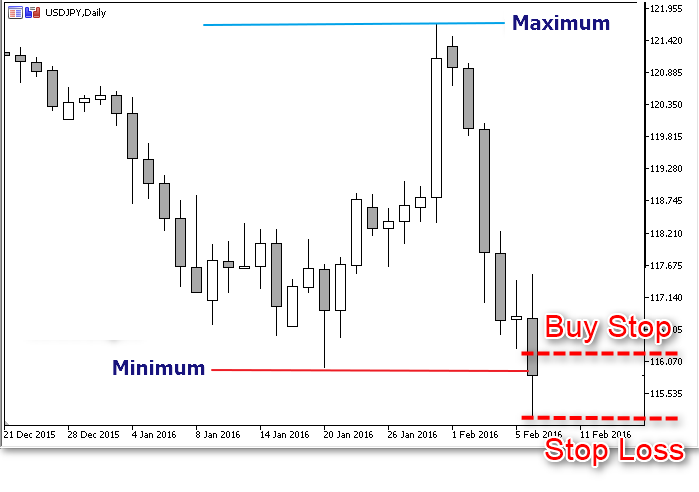```double d_Entry_Level = WRONG_VALUE; // 设置挂单的水平if(e_Signal == ENTRY_BUY) { // 对于买入挂单
// 检查是否可以设置订单:
d_Entry_Level = go_Channel.d_Low + gd_Entry_Offset; // 订单设置水平
// 与当前价格的距离不够
if(Log_Level > LOG_LEVEL_ERR)
PrintFormat("无法在 %s 水平设置止损买入挂单. Bid: %s Ask: %s StopLevel: %s",
DoubleToString(d_Entry_Level, _Digits),
DoubleToString(go_Tick.bid, _Digits),
DoubleToString(gd_Stop_Level, _Digits)
);

return; // 等待当前价格改变
}
} else {
// 检查是否可以设置订单:
d_Entry_Level = go_Channel.d_High - gd_Entry_Offset; // 订单设置水平
if(!fb_Is_Acceptable_Distance(d_Entry_Level, go_Tick.bid)) {
// 与当前价格的距离不够
if(Log_Level > LOG_LEVEL_ERR)
PrintFormat("无法在 %s 水平设置止损卖出挂单，Bid: %s Ask: %s StopLevel: %s",
DoubleToString(d_Entry_Level, _Digits),
DoubleToString(go_Tick.bid, _Digits),
DoubleToString(gd_Stop_Level, _Digits)
);

return; // 等待当前价格改变
}
}

```

```// 符合服务器要求的手数:double d_Volume = fd_Normalize_Lot(Trade_Volume);

// 设置挂单:

while(i_Try-- > 0) { // 尝试设置止损买入挂单
d_Volume,
d_Entry_Level,
_Symbol
)) { // 尝试成功
i_Try = -10; // 成功操作的标志
break;
}
// 失败
Sleep(gi_Connect_Wait); // 在下一次尝试前暂停
}
} else {
while(i_Try-- > 0) { // 尝试设置止损卖出挂单
d_Volume,
d_Entry_Level,
_Symbol
)) { // 尝试成功
i_Try = -10; // 成功操作的标志
break;
}
// 失败
Sleep(gi_Connect_Wait); // 在下一次尝试前暂停
}
}

if(i_Try == WRONG_VALUE) // 设置挂单失败
if(Log_Level > LOG_LEVEL_NONE) Print("设置挂单错误");

```

### 策略回测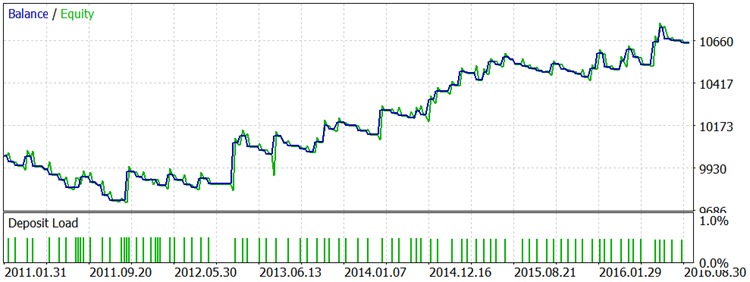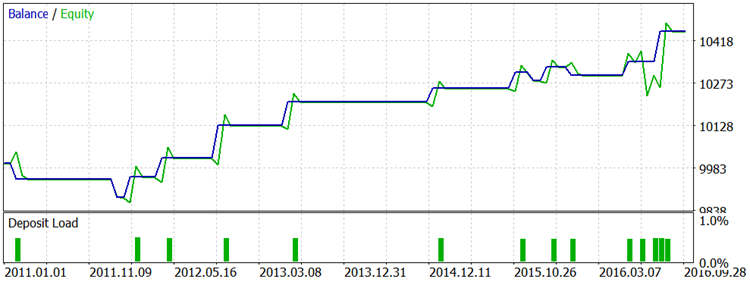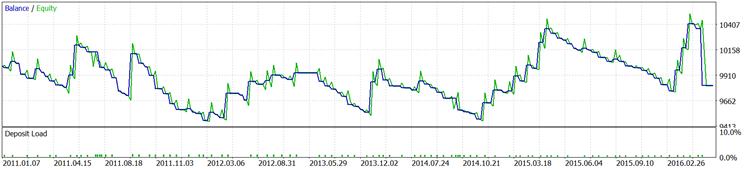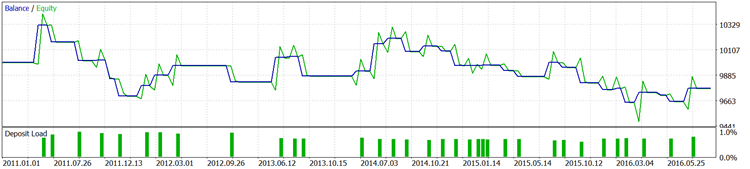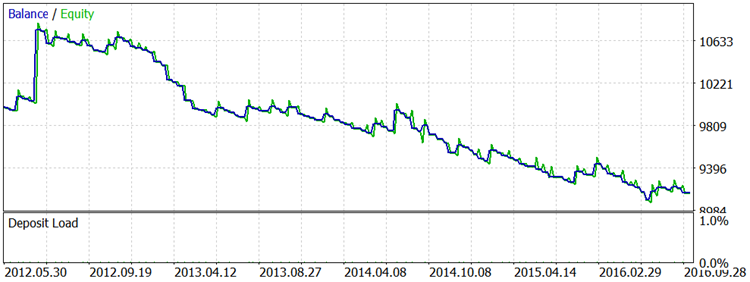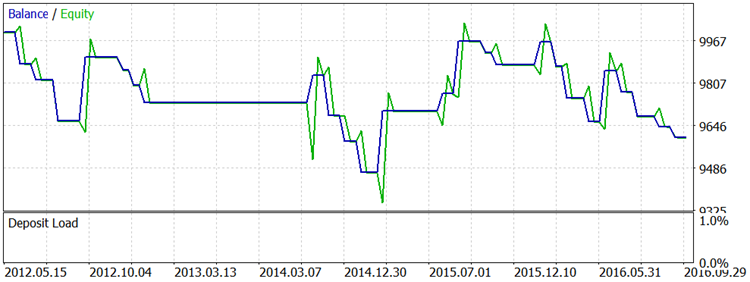### 结论

• 附件下载(1)：
•  2717.zip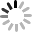7X15小时客户服务

EA配送

QQ或者微信发送
2小时内送达苏公网安备 32011302320766号

0